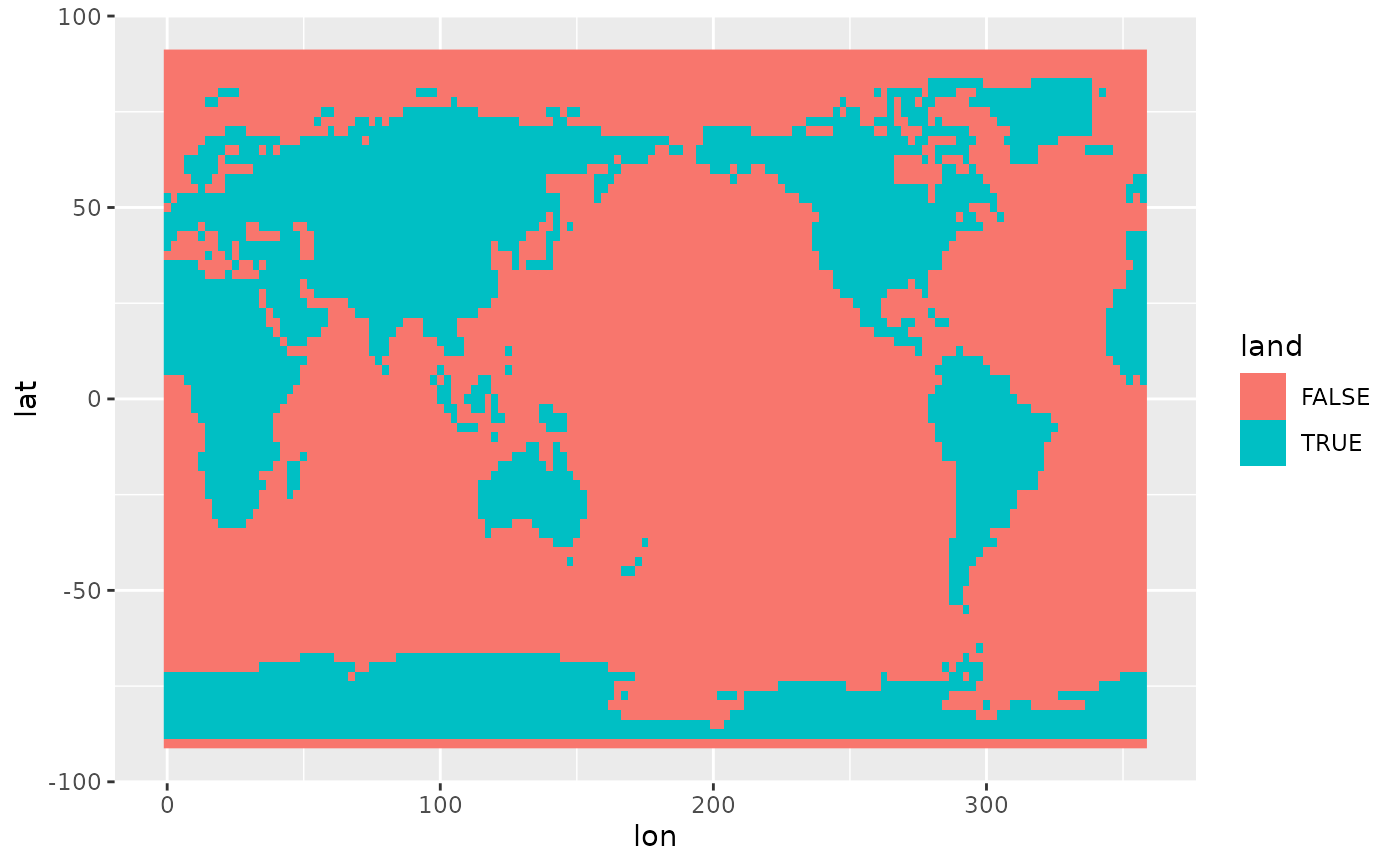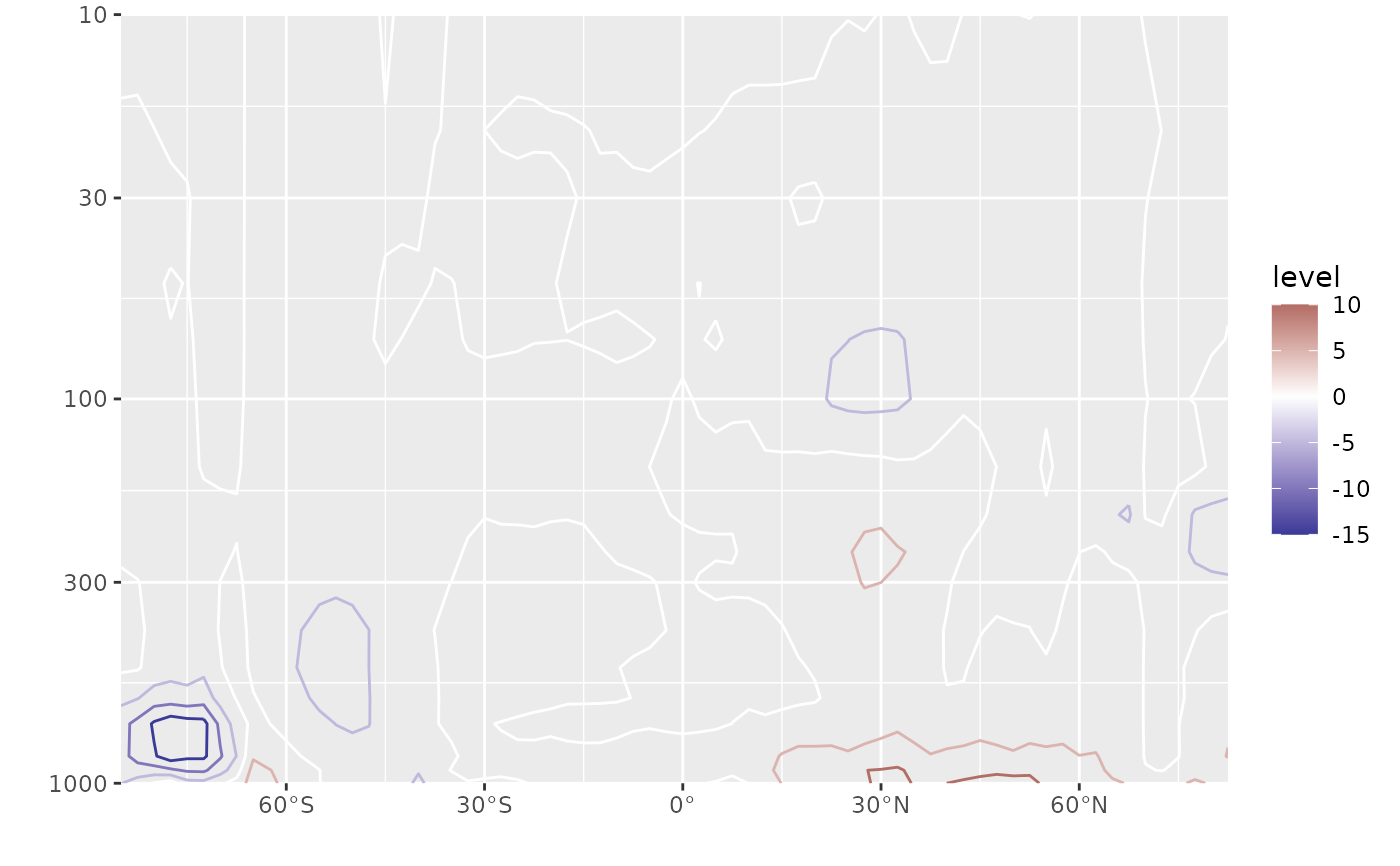MaskLand(lon, lat, mask = "world", wrap = c(0, 360))

## Arguments

lon

a vector of longitudes in degrees in 0-360 format

lat

a vector of latitudes in degrees

the name of the dataset (that will be load with map) for creating the mask

wrap

the longitude range to be used for a global mask

## Value

A logical vector of the same length as lat and lon where TRUE means that the point is inside one of the polygons making up the map. For a global map (the default), this means that the point is over land.

## Examples


mask <- temperature[lev == 1000, .(lon = lon, lat = lat, land = MaskLand(lon, lat))]
temperature <- temperature[mask, on = c("lon", "lat")]
library(ggplot2)

geom_raster(aes(fill = land))# Take the temperature difference between land and ocean
diftemp <- temperature[,
.(tempdif = mean(air[land == TRUE]) - mean(air[land == FALSE])),
by = .(lat, lev)]

ggplot(diftemp, aes(lat, lev)) +
geom_contour(aes(z = tempdif, color = after_stat(level))) +
scale_y_level() +
scale_x_latitude() +
scale_color_divergent()
#> Warning: Removed 136 rows containing non-finite values (stat_contour()).